## How to solve exponential equations

Examples of exponential equations.

$$2^{\red x} = 4 \\ 8^{\red{2x}} = 16 \\ \\ 16^{\red { x+1}} = 256 \\ \left( \frac{1}{2} \right)^{\red { x+1}} = 512$$

As you might've noticed, an exponential equation is just a special type of equation. It's an equation that has exponents that are $$\red{ variables}$$.

## Steps to Solve

There are different kinds of exponential equations. We will focus on exponential equations that have a single term on both sides. These equations can be classified into 2 types.

$$4^x = 4^9$$.

$$4^3 = 2^x$$.

$$\left( \frac{1}{4} \right)^x = 32$$ (Part II below)

## Part I. Solving Exponential Equations with Same Base

Solve: $$4^{x+1} = 4^9$$

Ignore the bases, and simply set the exponents equal to each other

$$x + 1 = 9$$

Solve for the variable

$$x = 9 - 1 \\ x = \fbox { 8 }$$

$$4^{x+1} = 4^9 \\ 4^{\red{8}+1} = 4^9$$ $$4^{\red{9} } = 4^9$$

## Exponential Equation Solver

Enter any exponential equation into the algebra solver below :

## II. Solving Exponential Equations with un -like bases

What do they look like.

$$\red 4^3 = \red 2^x$$ $$\red 9^x = \red { 81 }$$ $$\left( \red{\frac{1}{2}} \right)^{ x+1} = \red 4^3$$ $$\red 4^{2x} +1 = \red { 65 }$$

In each of these equations, the base is different. Our goal will be to rewrite both sides of the equation so that the base is the same.

Solve: $$4^{3} = 2^x$$Answer : They are both powers of 2

Rewrite equation so that both exponential expressions use the same base

$$\red 4^{3} = 2^x \\ (\red {2^2})^{3} = 2^x$$

Use exponents laws to simplify

$$(\red {2^2})^{3} = 2^x \\ (2^\red {2 \cdot 3 }) = 2^x \\ (2^\red 6 ) = 2^x$$

Solve like an exponential equation of like bases

$$(2^\red 6 ) = 2^x \\ x = \fbox{6}$$

Substitute $$\red 6$$ into the original equation to verify our work.

$$4^{3} = 2^{\red 6}$$ $$64 = 64$$

## Example with Negative Exponent

Unlike bases often involve negative or fractional bases like the example below. We are going to treat these problems like any other exponential equation with different bases--by converting the bases to be the same.

## Practice Problems ( un -like bases)

Solve the following exponential Equation: $$9^x = 81$$You can use either 3 or 9 . I will use 9.

$\\ 81 = \red 9 ^{\blue 2} \\ 9 = \red 9 ^{\blue 1} \\$

Substitute the rewritten bases into original equation

$$(\red 9^{\blue 1})^x = \red 9^{\blue 2}$$ 2 ) x =3 4 -->

$$(\red 9^{\blue 1})^x = \red 9^{\blue 2} \\ 9^{1 \cdot x } = 9 ^{2} \\ 9^{x } = 9 ^{2}$$ 2 x =3 4 -->

$$x = 2$$ -->

Solve the equation : $$4^{2x} +1 = 65$$

Rewrite this equation so that it looks like the other ones we solved. Isolate the exponential expression as follows:

$$4^{2x} +1 \red{-1} = 65\red{-1} \\ 4^{2x} = 64$$They are both powers of 2 and of 4 . You could use either base to solve this. I will use base 4

$\\ 64 = \red 4 ^{\blue 3} \\ 4 = \red 4 ^{\blue 1} \\$

$$4^{2x} = 64 \\ \red 4^{\blue{ 2x }} = \red 4^{\blue 3 }$$

$$\red 4^{\blue{ 2x }} = \red 4^{\blue 3 }$$ 2x = 4 3 -->

Not much to do this time :)

$$2x = 3 \\ x = \frac{3}{2}$$

Solve the exponential Equation : $$\left( \frac{1}{4} \right)^x = 32$$

Since these equations have different bases, follow the steps for unlike basesThey are both powers of 2

$\\ 32 = \red 2 ^{\blue 5} \\ \frac 1 4 = \red 2 ^{\blue {-2}} \\$

Rewrite as a negative exponent and substitute the rewritten bases into original equation

$$\left( \frac{1}{4} \right)^x = 32 \\ \left( \frac{1}{2^2} \right)^x = 32 \\ \left(\red 2 ^{\blue{-2}} \right)^x = \red 2^{\blue 5}$$

$$\left(\red 2 ^{\blue{-2}} \right)^x = \red 2^{\blue 5} \\ 2 ^{-2 \cdot x} = 2^5 \\ 2 ^{-2x} = 2^5$$

$$2 ^{-2x} = 2^5 \\ -2x = 5 \\ \frac{-2x}{-2} = \frac{5}{-2} \\ x = -\frac{5}{2}$$

Solve this exponential equation: $$\left( \frac{1}{9} \right)^x-3 = 24$$

$$\left( \frac{1}{9} \right)^x -3 \red{+3} =24\red{+3} \\ \left( \frac{1}{9} \right)^x=27$$They are both powers of 3 .

$\\ \frac 1 9 = \red 3 ^{\blue {-2}} \\ 27 = \red 3 ^{\blue 3} \\$

Rewrite as a negative exponent and substitute into original equation

$$\left( \frac{1}{9} \right)^x=27 \\ \left( \red{3^{-2}}\right)^x=\red{3^3 }$$

$$3^\red{{-2 \cdot x}} = 3^3 \\ 3^\red{{-2x}} = 3^3$$

$$-2x = 3 \\ x = \frac{3}{-2} \\ x = -\frac{3}{2}$$

Solve this exponential equation: $$\left( \frac{1}{25} \right)^{(3x -4)} -1 = 124$$

Rewrite this equation so that it looks like the other ones we solved--In other words, isolate the exponential expression as follows:

$$\left( \frac{1}{25} \right)^{(3x -4)} -1 \red{+1} = 124 \red{+1} \\ \left( \frac{1}{25} \right)^{(3x -4)} = 125$$They are both powers of 5 .

$\\ \frac { 1 } { 25 } = \red 5 ^{\blue {-2}} \\ 125 = \red 5 ^{\blue 3} \\$

$$\left( \frac{1}{25} \right)^{(3x -4)} = 125 \\ \left( \red{5^{-2}} \right)^{(3x -4)} = \red{5^3}$$

$$5^\red{{-2 \cdot (3x -4)}} = 5^3 \\ 5^\red{{(-6x + 8)}} = 5^3$$

$$-6x + 8 =3 \\ -6x = -5 \\ x = \frac{-5}{-6} \\ x = \frac{5}{6}$$

• Laws of Exponents
• Rules of Exponents

## Ultimate Math Solver (Free) Free Algebra Solver ... type anything in there!

Popular pages @ mathwarehouse.com.## How to Solve Exponents: A Step-by-Step Guide with ExamplesExponents, also known as powers or indices, are a fundamental concept in mathematics. It plays a crucial role in various fields, from basic arithmetic to advanced calculus. An exponent represents how many times a number, called the base, is multiplied by itself. To solve exponents involves understanding the properties of exponents, simplifying expressions, and performing operations with exponential terms. In this guide, we will walk you through the process of solving exponent problems using maths.ai as a teaching tool.

## Understanding Exponents

Before we delve into solving exponents problems, let’s review the basics of exponents.

An exponent is usually written as a superscript number next to the base. For example, in the expression 2^3, 2 is the base, and 3 is the exponent. This means that 2 is multiplied by itself three times: 2 * 2 * 2 = 8.

## Example 1: Simple Exponentiation

Let’s solve the problem: 4^2.

Step 1: Identify the base and the exponent.

Exponent: 2

Step 2: Apply the exponentiation rule.

4^2 = 4 * 4 = 16

## Exponent Properties

Understanding exponent properties is essential for solving more complex exponent problems.

## Property 1: Product of Powers

a^m * a^n = a^(m + n)

This property states that when you multiply two powers with the same base, you can add their exponents.

## Example 2: Product of Powers

Solve the problem: 3^4 * 3^2.

Step 1: Identify the base and the exponents.

Exponent 1: 4

Exponent 2: 2

Step 2: Apply the product of powers property.

3^4 * 3^2 = 3^(4 + 2) = 3^6 = 729

## Property 2: Quotient of Powers

a^m / a^n = a^(m – n)

This property states that when you divide two powers with the same base, you can subtract their exponents.

## Example 3: Quotient of Powers

Solve the problem: 5^7 / 5^4.

Exponent 1: 7

Exponent 2: 4

Step 2: Apply the quotient of powers property.

5^7 / 5^4 = 5^(7 – 4) = 5^3 = 125

## Property 3: Power of a Power

(a^m)^n = a^(m * n)

This property states that when you raise a power to another power, you can multiply the exponents.

## Example 4: Power of a Power

Solve the problem: (2^3)^4.

Exponent: 4

Step 2: Apply the power of a power property.

(2^3)^4 = 2^(3 * 4) = 2^12 = 4096

## Simplifying Exponential Expressions

Simplifying exponential expressions involves applying the properties of exponents to combine or rewrite terms for easier computation.

## Example 5: Simplifying Exponential Expressions

Simplify the expression: 2^5 * 2^8 / 2^3.

Exponent 1: 5, Exponent 2: 8, Exponent 3: 3

Step 2: Apply the properties of exponents.

2^5 * 2^8 / 2^3 = 2^(5 + 8 – 3) = 2^10 = 1024

## Operations with Exponential Terms

Performing operations with exponential terms involves applying the properties of exponents while considering addition, subtraction, multiplication, and division.

## Example 6: Operations with Exponential Terms

Solve the expression: (4^3 * 4^2) / 4^4 + 4^1.

Step 1: Identify the base and the exponents for each term.

Term 1: 4^3 * 4^2,Term 2: 4^4, Term 3: 4^1

Step 2: Simplify each term using the properties of exponents.

Term 1: 4^3 * 4^2 = 4^(3 + 2) = 4^5, Term 2: 4^4, Term 3: 4^1

Step 3: Combine the terms using addition and subtraction.

(4^5 * 4^4) / 4^4 + 4^1 = 4^5 + 4^1

Step 4: Calculate the final result.

4^5 + 4^1 = 1024 + 4 = 1028

Exponents are a fundamental concept in mathematics that allows us to represent repeated multiplication in a concise form. By understanding the properties of exponents, simplifying expressions, and performing operations with exponential terms, you can confidently tackle various exponent problems. Remember to apply the rules systematically, and when in doubt, use tools maths.ai to assist you in your journey towards mastering exponents. With practice and a clear grasp of exponent principles, you’ll be well-equipped to solve more complex maths problems that involve exponents.

## Related Posts## Understanding Vector: A Complete Guide## Mathematical Dimensions: An In-Depth Overview## Mastering Algebra: An In-Depth Manual

• Pingback: Solving Polynomials: A Step-by-Step Guide - maths.ai
• Pingback: Differentiation: Guide, with Examples and Methods - maths.ai
• Pingback: Mastering Algebra: An In-Depth Manual - maths.ai

Save my name, email, and website in this browser for the next time I comment.

• EXPLORE Tech Help Pro About Us Random Article Quizzes Request a New Article Community Dashboard This Or That Game Popular Categories Arts and Entertainment Artwork Books Movies Computers and Electronics Computers Phone Skills Technology Hacks Health Men's Health Mental Health Women's Health Relationships Dating Love Relationship Issues Hobbies and Crafts Crafts Drawing Games Education & Communication Communication Skills Personal Development Studying Personal Care and Style Fashion Hair Care Personal Hygiene Youth Personal Care School Stuff Dating All Categories Arts and Entertainment Finance and Business Home and Garden Relationship Quizzes Cars & Other Vehicles Food and Entertaining Personal Care and Style Sports and Fitness Computers and Electronics Health Pets and Animals Travel Education & Communication Hobbies and Crafts Philosophy and Religion Work World Family Life Holidays and Traditions Relationships Youth
• Browse Articles
• Learn Something New
• Quizzes Hot
• This Or That Game New
• Explore More
• Support wikiHow
• Education and Communications
• Mathematics
• Exponents and Logarithms

## How to Solve Exponential Equations

Last Updated: November 25, 2022 Fact Checked

Exponential equations may look intimidating, but solving them requires only basic algebra skills. Equations with exponents that have the same base can be solved quickly. In other instances, it is necessary to use logs to solve. Even this method, however, is simple with the aid of a scientific calculator.

## Equating Two Exponents with the Same Base## Equating an Exponent and a Whole Number## Using Logs for Terms without the Same Base## Expert Q&A## You Might Also Like• ↑ https://www.mathsisfun.com/definitions/exponent.html
• ↑ http://www.mathwarehouse.com/algebra/exponents/solve-exponential-equations-how-to.php
• ↑ https://courses.lumenlearning.com/intermediatealgebra/chapter/use-compound-interest-formulas/
• ↑ http://www.purplemath.com/modules/solvexpo.htm
• ↑ http://www.mathsisfun.com/algebra/exponents-logarithms.html
• ↑ https://tutorial.math.lamar.edu/classes/alg/solveexpeqns.aspxTo solve exponential equations with the same base, which is the big number in an exponential expression, start by rewriting the equation without the bases so you're left with just the exponents. Then, solve the new equation by isolating the variable on one side. To check your work, plug your answer into the original equation, and solve the equation to see if the two sides are equal. If they are, your answer is correct. To learn how to solve exponential equations with different bases, scroll down! Did this summary help you? Yes No

• Send fan mail to authors## Featured Articles## Trending Articles## Watch Articles• Do Not Sell or Share My Info
• Not Selling Info

wikiHow Tech Help Pro:

The exponent of a number says how many times to use the number in a multiplication.

In 8 2 the "2" says to use 8 twice in a multiplication, so 8 2 = 8 × 8 = 64

In words: 8 2 could be called "8 to the power 2" or "8 to the second power", or simply "8 squared"

## Example: 5 3 = 5 × 5 × 5 = 125

• In words: 5 3 could be called "5 to the third power", "5 to the power 3" or simply "5 cubed"

## Example: 2 4 = 2 × 2 × 2 × 2 = 16

• In words: 2 4 could be called "2 to the fourth power" or "2 to the power 4" or simply "2 to the 4th"

Exponents make it easier to write and use many multiplications

Example: 9 6 is easier to write and read than 9 × 9 × 9 × 9 × 9 × 9

You can multiply any number by itself as many times as you want using exponents.

So in general :

## Another Way of Writing It

Sometimes people use the ^ symbol (above the 6 on your keyboard), as it is easy to type.

Example: 2^4 is the same as 2 4

• 2^4 = 2 × 2 × 2 × 2 = 16

## Negative Exponents

Negative? What could be the opposite of multiplying? Dividing!

So we divide by the number each time, which is the same as multiplying by 1 number

Example: 8 -1 = 1 8 = 0.125

We can continue on like this:

Example: 5 -3 = 1 5 × 1 5 × 1 5 = 0.008

But it is often easier to do it this way:

5 -3 could also be calculated like:

1 5 × 5 × 5 = 1 5 3 = 1 125 = 0.008

More Examples:

## What if the Exponent is 1, or 0?

It all makes sense.

If you look at that table, you will see that positive, zero or negative exponents are really part of the same (fairly simple) pattern:

## Solver Title## Generating PDF...

• Pre Algebra Order of Operations Factors & Primes Fractions Long Arithmetic Decimals Exponents & Radicals Ratios & Proportions Percent Modulo Mean, Median & Mode Scientific Notation Arithmetics
• Algebra Equations Inequalities System of Equations System of Inequalities Basic Operations Algebraic Properties Partial Fractions Polynomials Rational Expressions Sequences Power Sums Interval Notation Pi (Product) Notation Induction Logical Sets Word Problems
• Pre Calculus Equations Inequalities Simultaneous Equations System of Inequalities Polynomials Rationales Complex Numbers Polar/Cartesian Functions Arithmetic & Comp. Coordinate Geometry Plane Geometry Solid Geometry Conic Sections Trigonometry
• Calculus Derivatives Derivative Applications Limits Integrals Integral Applications Integral Approximation Series ODE Multivariable Calculus Laplace Transform Taylor/Maclaurin Series Fourier Series Fourier Transform
• Functions Line Equations Functions Arithmetic & Comp. Conic Sections Transformation
• Linear Algebra Matrices Vectors
• Trigonometry Identities Proving Identities Trig Equations Trig Inequalities Evaluate Functions Simplify
• Statistics Mean Geometric Mean Quadratic Mean Average Median Mode Order Minimum Maximum Probability Mid-Range Range Standard Deviation Variance Lower Quartile Upper Quartile Interquartile Range Midhinge Standard Normal Distribution
• Physics Mechanics
• Chemistry Chemical Reactions Chemical Properties
• Finance Simple Interest Compound Interest Present Value Future Value
• Economics Point of Diminishing Return
• Conversions Radical to Exponent Exponent to Radical To Fraction To Decimal To Mixed Number To Improper Fraction Radians to Degrees Degrees to Radians Hexadecimal Scientific Notation Distance Weight Time
• Pre Algebra
• One-Step Subtraction
• One-Step Multiplication
• One-Step Division
• One-Step Decimals
• Two-Step Integers
• Two-Step Multiply/Divide
• Two-Step Fractions
• Two-Step Decimals
• Multi-Step Integers
• Multi-Step with Parentheses
• Multi-Step Rational
• Multi-Step Fractions
• Multi-Step Decimals
• Solve by Factoring
• Completing the Square
• Logarithmic
• Exponential
• Rational Roots
• Floor/Ceiling
• Equation Given Roots
• Newton Raphson
• Substitution
• Elimination
• Cramer's Rule
• Gaussian Elimination
• System of Inequalities
• Perfect Squares
• Difference of Squares
• Difference of Cubes
• Sum of Cubes
• Polynomials
• Distributive Property
• FOIL method
• Perfect Cubes
• Binomial Expansion
• Negative Rule
• Product Rule
• Quotient Rule
• Expand Power Rule
• Fraction Exponent
• Exponent Rules
• Exponential Form
• Logarithmic Form
• Absolute Value
• Rational Number
• Powers of i
• Partial Fractions
• Is Polynomial
• Standard Form
• Complete the Square
• Synthetic Division
• Linear Factors
• Rationalize Denominator
• Rationalize Numerator
• Identify Type
• Convergence
• Interval Notation
• Pi (Product) Notation
• Boolean Algebra
• Truth Table
• Mutual Exclusive
• Cardinality
• Caretesian Product
• Age Problems
• Distance Problems
• Cost Problems
• Investment Problems
• Number Problems
• Percent Problems
• Multiplication/Division
• Dice Problems
• Coin Problems
• Card Problems
• Pre Calculus
• Linear Algebra
• Trigonometry
• Conversions## Most Used Actions

Number line.

• 2^{x}\cdot2^{y}
• (x^{3})^{5}
• \frac{x^{12}}{x^{-2}}
• x^{2}\cdot x^{3}

exponents-calculator

• Middle School Math Solutions – Inequalities Calculator Next up in our Getting Started maths solutions series is help with another middle school algebra topic - solving... Read More
• Math Article
• Exponents Powers

## Exponents And Powers

Exponents and powers are ways used to represent very large numbers or very small numbers in a simplified manner. For example, if we have to show 3 x 3 x 3 x 3 in a simple way, then we can write it as 3 4 , where 4 is the exponent and 3 is the base. The whole expression 3 4 is said to be power. Also learn  the  laws of exponents here.

Basically, power is an expression that shows repeated multiplication of the same number or factor. The value of the exponent is based on the number of times the base is multiplied to itself. See of the examples here:

## What is Exponent?

An exponent of a number, represents the number of times the number is multiplied to itself. If 8 is multiplied by itself for n times, then, it is represented as:

8 x 8 x 8 x 8 x …..n times = 8 n

The above expression, 8 n , is said as 8 raised to the power n. Therefore, exponents are also called power or sometimes indices.

• 2 x 2 x 2 x 2 = 2 4
• 5 x 5 x 5 = 5 3
• 10 x 10 x 10 x 10 x 10 x 10 = 10 6

## General Form of Exponents

The exponent is a simple but powerful tool. It tells us how many times a number should be multiplied by itself to get the desired result. Thus any number ‘a’ raised to power ‘n’ can be expressed as: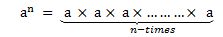Here a is any number and n is a natural number.

a n  is also called the nth power of a .

‘a’ is the base and ‘n’ is the exponent or index or power.

‘a’ is multiplied ‘n’ times, and thereby exponentiation is the shorthand method of repeated multiplication.

• Exponents and Powers for Class 7
• Exponents And Powers for Class 8

## Laws of Exponents

The laws of exponents are demonstrated based on the powers they carry.

• Bases – multiplying the like ones – add the exponents and keep the base same. (Multiplication Law)
• Bases – raise it with power to another – multiply the exponents and keep the base same.
• Bases – dividing the like ones – ‘Numerator Exponent – Denominator Exponent’ and keep the base same. (Division Law)

Let ‘a’ is any number or integer (positive or negative) and ‘m’,  ‘n’ are positive integers, denoting the power to the bases, then;

## Multiplication Law

As per the multiplication law of exponents, the product of two exponents with the same base and different powers equals to base raised to the sum of the two powers or integers.

a m  × a n   = a m+n

## Division Law

When two exponents having same bases and different powers are divided, then it results in base raised to the difference between the two powers.

a m ÷ a n   = a m  / a n   = a m-n

## Negative Exponent Law

Any base if has a negative power, then it results in reciprocal but with positive power or integer to the base.

a -m    = 1/a m

## Rules of Exponents

The rules of exponents are followed by the laws. Let us have a look at them with a brief explanation.

Suppose ‘a’ & ‘b’ are the integers and ‘m’ & ‘n’ are the values for powers, then the rules for exponents and powers are given by:

i) a 0  = 1

As per this rule, if the power of any integer is zero, then the resulted output will be unity or one.

Example: 5 0 = 1

ii) (a m ) n  = a( mn )

‘a’ raised to the power ‘m’ raised to the power ‘n’ is equal to ‘a’ raised to the power product of ‘m’ and ‘n’.

Example: (5 2 ) 3  = 5 2 x 3

iii) a m  × b m  =(ab) m

The product of ‘a’ raised to the power of ‘m’ and ‘b’ raised to the power ‘m’ is equal to the product of ‘a’ and ‘b’ whole raised to the power ‘m’.

Example:  5 2  × 6 2  =(5 x 6) 2

iv) a m /b m  = (a/b) m

The division of ‘a’ raised to the power ‘m’ and ‘b’ raised to the power ‘m’ is equal to the division of ‘a’ by ‘b’ whole raised to the power ‘m’.

Example:  5 2 /6 2  = (5/6) 2

## Solved Questions

Example 1: Write 7 x 7 x 7 x 7 x 7 x 7 x 7 x 7 in exponent form.

In this problem 7s are written 8 times, so the problem can be rewritten as an exponent of 8.

7 x 7 x 7 x 7 x 7 x 7 x 7 x 7 = 7 8 .

Example 2 : Write below problems like exponents:

• 3 x 3 x 3 x 3 x 3 x 3
• 7 x 7 x 7 x 7 x 7
• 10 x 10 x 10 x 10 x 10 x 10 x 10
• 3 x 3 x 3 x 3 x 3 x 3 = 3 6
• 7 x 7 x 7 x 7 x 7 = 7 5
• 10 x 10 x 10 x 10 x 10 x 10 x 10 = 10 7

Example 3: Simplify 25 3 /5 3

Using Law: a m /b m  = (a/b) m

25 3 /5 3   can be written as (25/5) 3   = 5 3  = 125.

## Exponents and Powers Applications

Scientific notation uses the power of ten expressed as exponents, so we need a little background before we can jump in. In this concept, we round out your knowledge of exponents, which we studied in previous classes.

The distance between the Sun and the Earth is 149,600,000 kilometres. The mass of the Sun is 1,989,000,000,000,000,000,000,000,000,000 kilograms. The age of the Earth is 4,550,000,000 years. These numbers are way too large or small to memorize in this way.  With the help of exponents and powers, these huge numbers can be reduced to a very compact form and can be easily expressed in powers of 10.

Now, coming back to the examples we mentioned above, we can express the distance between the Sun and the Earth with the help of exponents and powers as following:

Distance between the Sun and the Earth 149,600,000 = 1.496× 10 × 10 × 10 × 10 × 10× 10 × 10 = 1.496× 10 8 kilometers.

Mass of the Sun: 1,989,000,000,000,000,000,000,000,000,000 kilograms = 1.989 × 10 30  kilograms.

Age of the Earth:  4,550,000,000 years = 4. 55× 10 9  years

## Video Lesson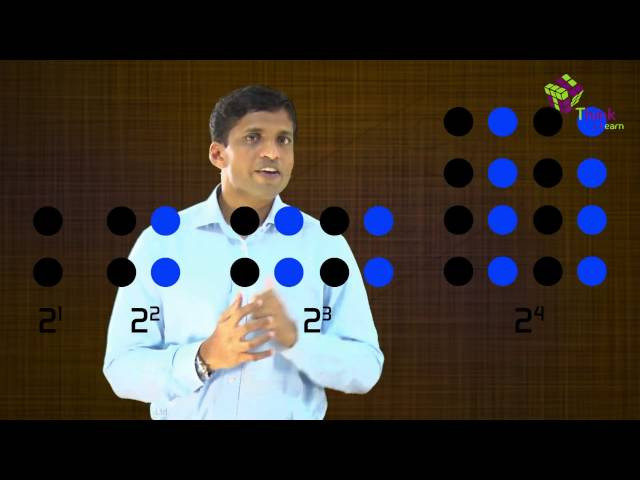## Frequently Asked Questions – FAQs

What are powers and exponents, are powers and exponents the same, what are the examples of exponents, what are the rules of exponents, what are negative exponents, what do we get if the exponent is 1 or 0, leave a comment cancel reply.

Request OTP on Voice Call

Post My Comment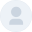Hi and thanks for this video• Share Share

Register with byju's & watch live videos.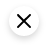If you're seeing this message, it means we're having trouble loading external resources on our website.

If you're behind a web filter, please make sure that the domains *.kastatic.org and *.kasandbox.org are unblocked.

## Course: Algebra 2   >   Unit 6

Evaluating fractional exponents.

• Evaluating fractional exponents: negative unit-fraction
• Evaluating fractional exponents: fractional base
• Evaluating quotient of fractional exponents
• Evaluating mixed radicals and exponents

## Want to join the conversation?

• Upvote Button navigates to signup page
• Downvote Button navigates to signup page
• Flag Button navigates to signup page## Video transcript#### IMAGES

1. How to Solve Algebraic Problems With Exponents: 8 Steps2. Solving Exponential Equations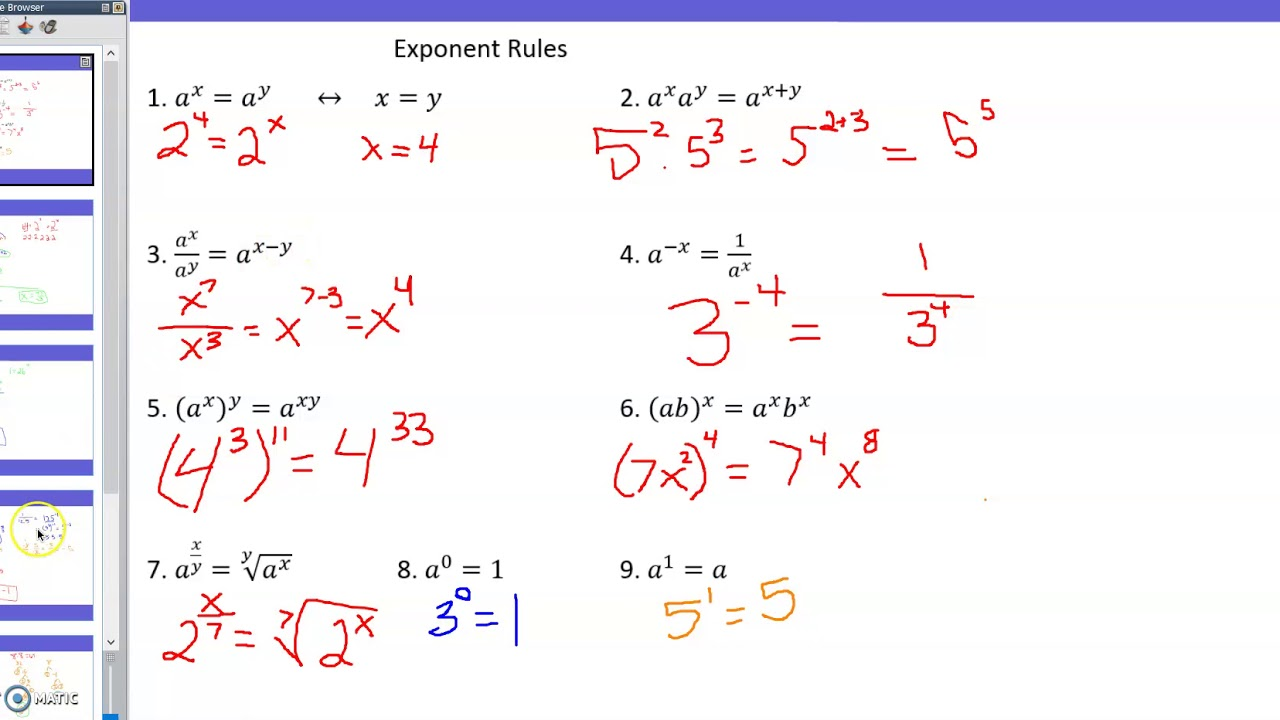3. How to Solve for Exponents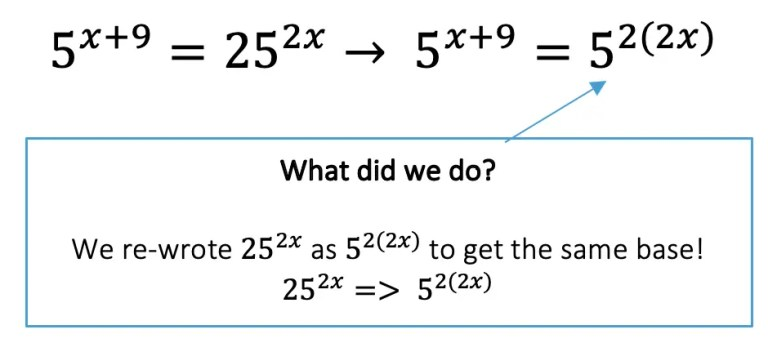4. How to Solve Exponents5. How to Solve for Exponents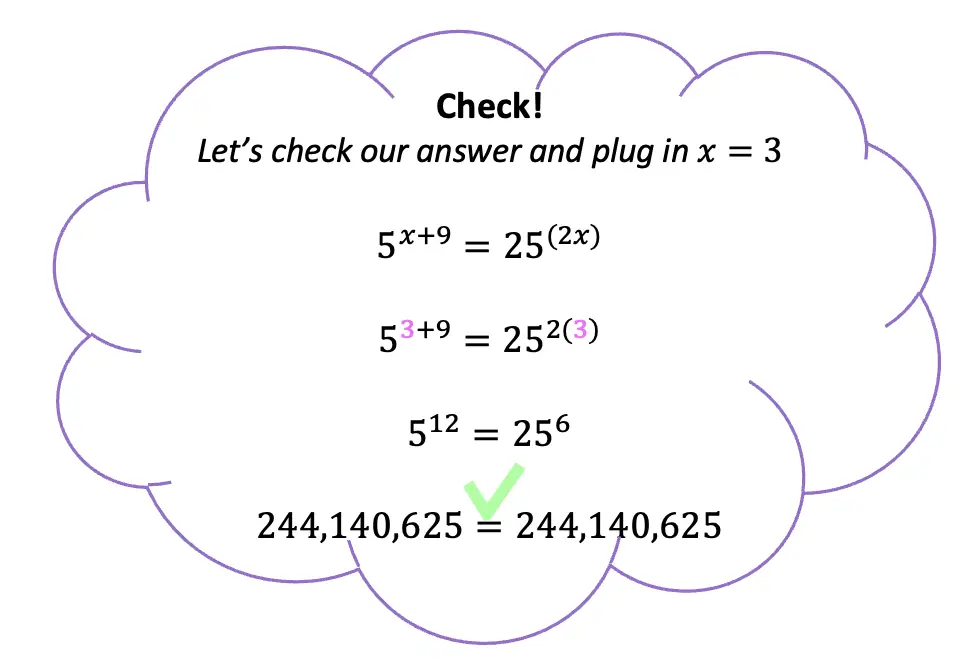6. How to Solve for Exponents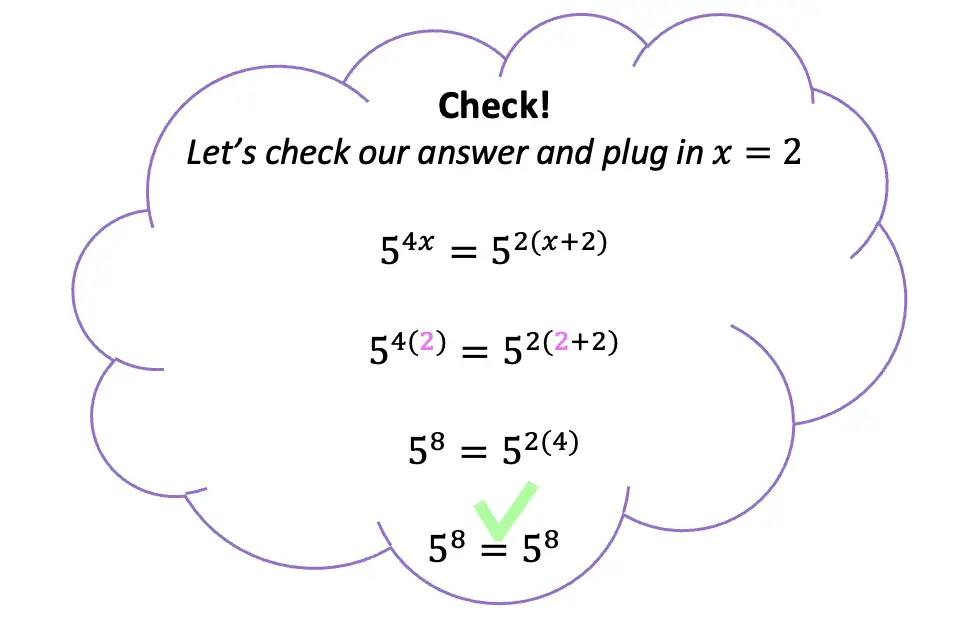#### VIDEO

1. How to Solve Exponents

2. Solve Easily Maths Problems

3. Easily Solve Maths Problems

4. Solve Easily Maths Problems

5. A Nice Exponents Multiplication Problem

6. A Nice Exponents Problem

1. Solving exponential equations using exponent properties

Solving exponential equations using exponent properties Google Classroom About Transcript Sal solves equations like 26^ (9x+5) = 1 and 2^ (3x+5) = 64^ (x-7). Questions Tips & Thanks Want to join the conversation? Sort by: Top Voted letzkusb000 5 years ago

2. How to solve exponential equations

Step 1 Ignore the bases, and simply set the exponents equal to each other x + 1 = 9 x + 1 = 9 Step 2 Solve for the variable x = 9 − 1 x = 8 x = 9 − 1 x = 8 Check We can verify that our answer is correct by substituting our value back into the original equation . . 4x+1 = 49 48+1 = 49 4 x + 1 = 4 9 4 8 + 1 = 4 9 49 = 49 4 9 = 4 9

3. 3 Ways to Solve Exponents

Method 1 Solving Basic Exponents Download Article 1 Learn the correct words and vocabulary for exponent problems. When you have an exponent, like , you have two simple parts. The bottom number, here a 2, is the base. The number it is raised to, here a 3, is known as the exponent or power.

4. How to Solve Exponents: A Step-by-Step Guide with Examples

To solve exponents involves understanding the properties of exponents, simplifying expressions, and performing operations with exponential terms. In this guide, we will walk you through the process of solving exponent problems using maths.ai as a teaching tool. Understanding Exponents

5. Algebra

There are two methods for solving exponential equations. One method is fairly simple but requires a very special form of the exponential equation. The other will work on more complicated exponential equations but can be a little messy at times. Let's start off by looking at the simpler method.

6. Microsoft Math Solver

Type a math problem Solve trigonometry Get step-by-step explanations See how to solve problems and show your work—plus get definitions for mathematical concepts Graph your math problems Instantly graph any equation to visualize your function and understand the relationship between variables Practice, practice, practice

7. How to Solve Algebraic Problems With Exponents: 8 Steps

Method 1 Solving a Problem with Exponents Download Article 1 Address the order of operations. Just like any problem in mathematics, an algebraic problem must be completed by the order of operations.

8. 3 Ways to Solve Exponential Equations

1 Determine whether the two exponents have the same base. The base is the big number in an exponential expression.  You can only use this method when you are presented with an equation that has an exponent on either side, and each exponent has the same base. For example,

9. Expressions with exponents

Algebra basics Unit 6: Expressions with exponents 1,200 possible mastery points Mastered Proficient Familiar Attempted Not started Quiz Unit test About this unit Expand your algebra superpowers by introducing exponents! Let's build our toolkit that allows us to manipulate exponents algebraically. Exponent properties intro Learn

10. Solve exponential equations using exponent properties

You might need: Calculator Solve the exponential equation for x . 9 3 x − 10 = ( 1 81) 1 6 x = Show Calculator Stuck? Review related articles/videos or use a hint. Report a problem Do 4 problems Learn for free about math, art, computer programming, economics, physics, chemistry, biology, medicine, finance, history, and more.

11. Exponents

The exponent of a number says how many times to use the number in a multiplication. In 82 the "2" says to use 8 twice in a multiplication, so 82 = 8 × 8 = 64. In words: 8 2 could be called "8 to the power 2" or "8 to the second power", or simply "8 squared". Some more examples:

12. Exponents

Learn about exponents using our free math solver with step-by-step solutions. Skip to main content. Microsoft ... Solve Equations ... Type a math problem. Solve. Examples. x \cdot x^2 \cdot 3x. n^4 \cdot 2n^2 \cdot n^5 (2a \cdot 3b^2)^2 \cdot c \cdot (2bc^3)^3 ...

13. Exponents Calculator

Free Exponents Calculator - Simplify exponential expressions using algebraic rules step-by-step

Algebra 2 12 units · 113 skills. Unit 1 Polynomial arithmetic. Unit 2 Complex numbers. Unit 3 Polynomial factorization. Unit 4 Polynomial division. Unit 5 Polynomial graphs. Unit 6 Rational exponents and radicals. Unit 7 Exponential models. Unit 8 Logarithms.

15. Multiplying & dividing powers (integer exponents)

David Severin. 2 years ago. The rule for dividing same bases is x^a/x^b=x^ (a-b), so with dividing same bases you subtract the exponents. In the case of the 12s, you subtract -7- (-5), so two negatives in a row create a positive answer which is where the +5 comes from. In the x case, the exponent is positive, so applying the rule gives x^ (-20 ...

16. Solving Problems Involving Exponents

Review how to solve 12 different problems involving exponents. The problems include working with exponents in base ten, simplifying exponential expressions,...

17. Exponent example 1 (video)

Try the practice problems on paper so your brain will make the logical connections of the symbols and results! Comment Button navigates to signup page (8 votes) ... Exponents are just repeated multiplication. 5 to power 2 is 5 time itself twice, or 5x5. 5 to power 3 is 5 times itself thrice, or 5x5x5. 5x5=25, and 25x2=125. The value of 5 to ...

18. Algebra

Here is a set of practice problems to accompany the Solving Exponential Equations section of the Exponential and Logarithm Functions chapter of the notes for Paul Dawkins Algebra course at Lamar University.

19. Solving Exponential Equations

This algebra video tutorial explains how to solve exponential equations using basic properties of logarithms and natural logs. Examples include factoring tr...

20. Solve for Exponents Calculator

How to solve for exponents For x n = y; solve for n by taking the log of both sides of the equation: For: x n = y Take the log of both sides: log x n = log y By identity we get: n ⋅ log x = log y Dividing both sides by log x: n = log y log x Find the exponent of a number

21. 1.5: Rules of Exponents and Scientific Notation

Expressions with negative exponents in the denominator can be rewritten as expressions with positive exponents in the numerator: $$\frac { 1 } { x ^ { - m } } = x ^ { m }$$. Take care to distinguish negative coefficients from negative exponents. Scientific notation is particularly useful when working with numbers that are very large or very small.

22. Exponents and Powers (Rules and Solved Examples)

Example 1: Write 7 x 7 x 7 x 7 x 7 x 7 x 7 x 7 in exponent form. Solution: In this problem 7s are written 8 times, so the problem can be rewritten as an exponent of 8. 7 x 7 x 7 x 7 x 7 x 7 x 7 x 7 = 78. Example 2: Write below problems like exponents: 3 x 3 x 3 x 3 x 3 x 3. 7 x 7 x 7 x 7 x 7.

23. Evaluating fractional exponents

7776 = 6^5 (rather than going through factoring, I did 7776^(1/5) in calculator), so squaring we end up with (-6)^2 which ends up as 36. With a negative exponent, this causes the expression to reciprocate and change exponent to positive, so start with 1/(4096)^(5/6) = 1/4^5 = 1/1024.

24. Solved Use the like-bases property and exponents to solve

Solution:-. Given:-. View the full answer Step 2. Unlock. Answer. Unlock. Previous question Next question. Transcribed image text: Use the like-bases property and exponents to solve the equation 6(5)x +27=777.